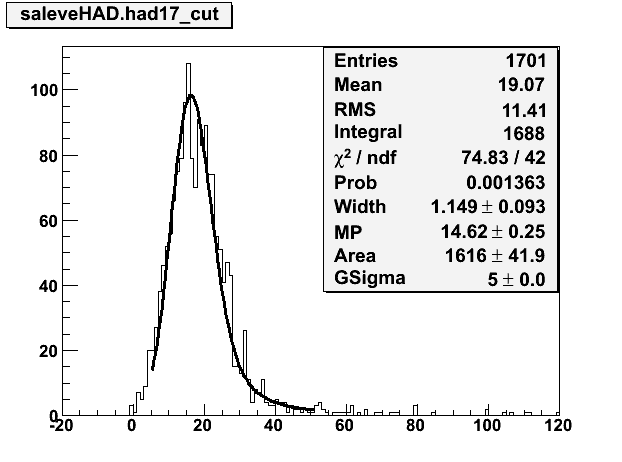# How to find peak on the langaus fit?

hi,
How can i find the peak on the langaus fit?sample plot attached.
Shall i send you any script which i used???-do you want to find an initial estimate for the peak . if yes see example using TSpectrum at \$ROOTSYS/tutorials/spectrum/peaks.C
-do you want to retrive the value of the “Most Probable Value” computed by the fit?
In this case, youe TF1::GetParameter

Rene

Dear Rene,

If you look at the plot, the distribution Gaus weighted. I mean GSigma=5.00 which is much greater than Landau one. So my problem is to find the peak of gaussian part on the langaus fit. Would it possible?

Cheers,
Tugba

I am still not sure to understand your question. It is clear that when fitting the same distribution with a gauss or landau the sigma of the gauss will be much bigger than with Landau.
For the meaning of the Landau parameters, see the doc of TMath::Landau at:

root.cern.ch/root/html/TMath.html#TMath:Landau

Rene